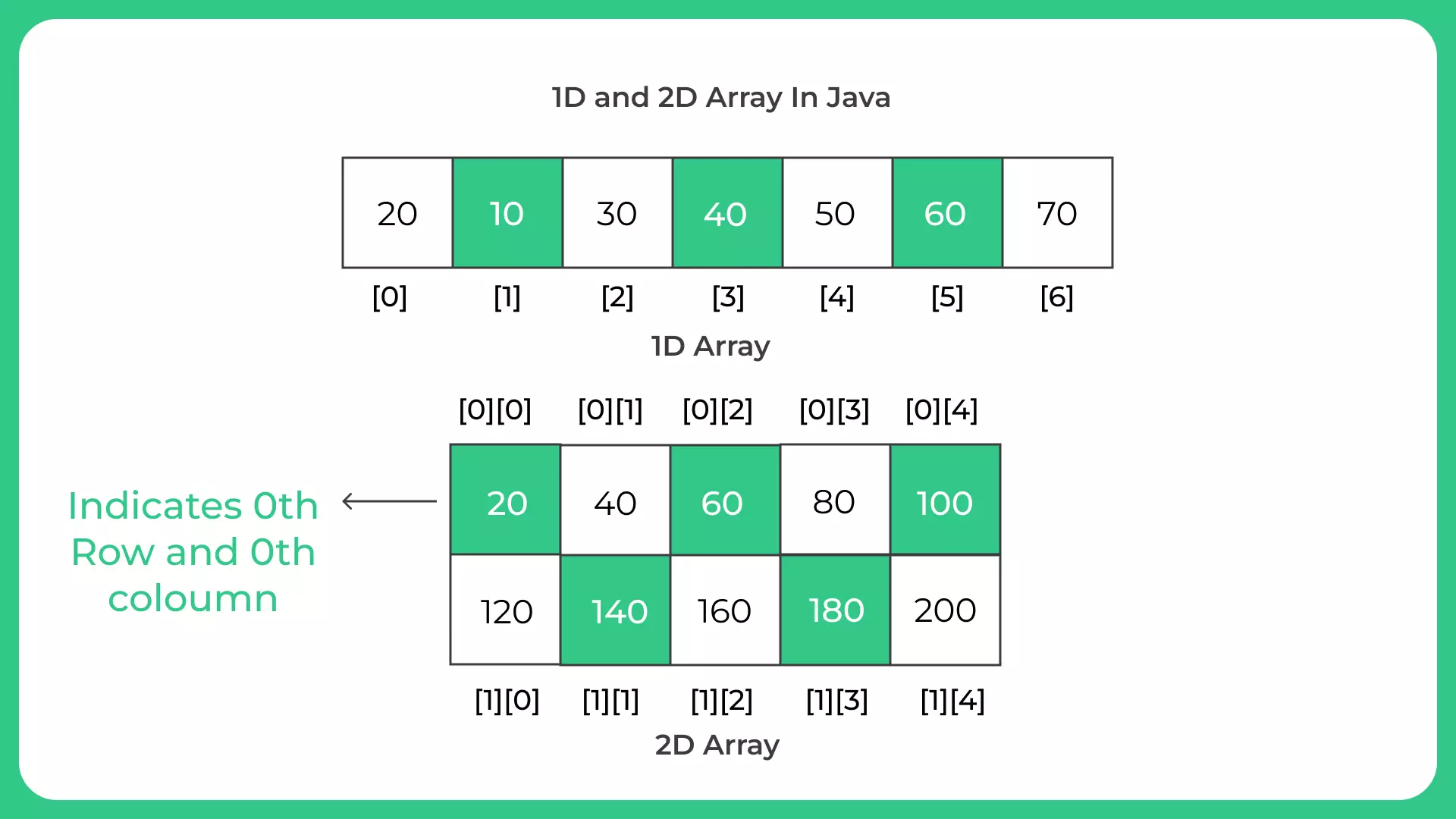# 1D and 2D Arrays In Java

## 1D and 2D Arrays In Java

Arrays are a fundamental class of data structure used in computer programming that enable effective data management and storage. They are a collection of linked data items that are stored near to one another in memory. Both one-dimensional (1D) and two-dimensional (2D) arrays are supported in the programming language java. We will discuss the differences between 1D & 2D arrays in Java in this page. Before going into the distinctions, you should be familiar with 1D and 2D arrays as well as their benefits and drawbacks.## 1D Array

Array is a data structure that is used to store variables that are of similar data types at contiguous locations. The main advantage of the array is random access and cache.

• A one-dimensional (1D) array is a collection of identical data type items that is linearly ordered. All of the components of a 1D array are stored in a single, continuous region of memory, and each component is reachable by its index. The first element of an array is always indexed 0, and the last member is always indexed n-1, where n is the array’s size.In Java language, int[] numbers; declares an array called numbers. Then, we can allocate memory for that array using ‘new’ keyword as follows.

• numbers= new int;

This array is capable of storing 10 integer values. We can combine the above two statements together and write as follows.

• int numbers = new int;

Below is an example of assigning values to the array.

• numbers ={1,2,3,4,5,6,7,8,9,10};

The starting index of an array is 0. Therefore, the element in the 0th index is 1. The element in the 1st index is 2. The element in the 2nd index is 3, etc. The index of the final element is 9.

If the programmer wants to store number 50 on the 2nd index, he can write the statement as follows.

• numbers = 50;

### Related Banners

Get PrepInsta Prime & get Access to all 200+ courses offered by PrepInsta in One Subscription

## 2D Array

Identical data-type components are grouped together and placed in a grid-like arrangement to form a 2D array. In essence, it is an array of arrays, where each array represents a row of a grid. The elements of a 2D array are stored in a single, continuous area of memory, and each element is accessible via its row and column indices. The first index stands in for the row number, while the second index stands in for the column number.

For example, int[][] numbers; declares a 2D arrays.

• numbers = new int ;

The above statement allocates memory for a 2D array of 2 rows and 3 columns. We can combine the above two statements together and write the statement as follows.

• int[][] numbers = new int;

Below is an example of assigning values to the 2D array.

• int[][] numbers = { {10,20,30}, {50,60,70}};

Similar to a 1D array, the starting index of the 2D array is also 0. This array has two rows and three columns. The indexes of the rows are 0 and 1 while the indexes of columns are 0, 1 and 2. The element 10 is in the 0th row 0th column position. Number 20 is in the 0th row, 1st column position. Number 70 is in 1st row, 2nd column position.

• numbers = 50;

Above statement assigns number 50 to 1st row, 2nd column position.

## Get over 200+ course One Subscription

Courses like AI/ML, Cloud Computing, Ethical Hacking, C, C++, Java, Python, DSA (All Languages), Competitive Coding (All Languages), TCS, Infosys, Wipro, Amazon, DBMS, SQL and others